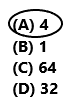# Texas Go Math Grade 5 Lesson 6.4 Answer Key Divide Fractions and Whole Numbers

Refer to our Texas Go Math Grade 5 Answer Key Pdf to score good marks in the exams. Test yourself by practicing the problems from Texas Go Math Grade 5 Lesson 6.4 Answer Key Divide Fractions and Whole Numbers.

## Texas Go Math Grade 5 Lesson 6.4 Answer Key Divide Fractions and Whole Numbers

Investigate

Materials; fraction strips

A. Mia walks a 2-mile fitness trail. She stops to exercise every $$\frac{1}{5}$$ mile. How many times does Mia stop to exercise?
Draw a number line from 0 to 2. Divide the number line into fifths. Label each fifth on your number line.Skip count by fifths from 0 to 2 to find 2 ÷ $$\frac{1}{5}$$.
There are ________ one-fifths in 2 wholes.

You can use the relationship between multiplication and division to explain and check your solution.

Record and check the quotient.
2 ÷ $$\frac{1}{5}$$ = ________ because ________ × $$\frac{1}{5}$$ = 2.
So, Mia stops to exercise ________ times.
We have to draw a number line from 0 to 2. Divide the number line into fifths. We have to label each fifth on our number line.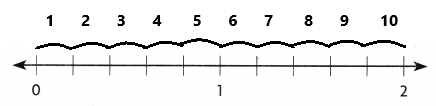Skip count by fifths from 0 to 2 to find 2 ÷ (1/5).
There are 10 one-fifths in 2 wholes.
We can use the relationship between multiplication and division to explain and check our solution.
Record and check the quotient.
2 ÷ (1/5) =10 because 10 × $$\frac{1}{5}$$ = 2.
So, Mia stops to exercise 10 times.

B. Roger has 2 yards of string. He cuts the string into pieces that are $$\frac{1}{3}$$ yard long. How many pieces of string does Roger have?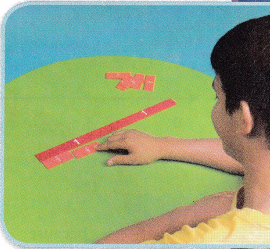Model 2 using 2 whole fraction strips.
Then place enough $$\frac{1}{3}$$ strips to fit exactly under the 2 wholes. There are _________ one-third-size pieces in 2 wholes.
Record and check the quotient.
2 ÷ $$\frac{1}{3}$$ = _________ because _________ × $$\frac{1}{3}$$ = 2.
So, Roger has ________ pieces of string.
Roger has 2 yards of string. He cuts the string into pieces that are 1/3 yard long.
Model 2 using 2 whole fraction strips.
Then place enough 1/3 strips to fit exactly under the 2 wholes. There are 6 one-third-size pieces in 2 wholes.
Record and check the quotient.
2 ÷(1/3) = 6 because 6 × 1/3 = 2.
So, Roger has 6 pieces of string.

Make Connections

You can use fraction strips to divide a fraction by a whole number.

Calla shares half of a package of clay equally among herself and each of 2 friends. What fraction of the whole package of clay will each friend get?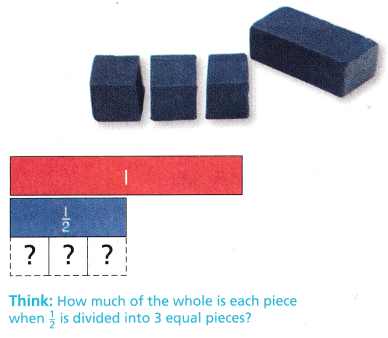STEP 1: Place a strip under a $$\frac{1}{2}$$-whole strip to show the $$\frac{1}{2}$$ package of clay.

STEP 2: Find 3 fraction strips, all with the same denominator, that fit exactly under the $$\frac{1}{2}$$ strip.
Each piece is __________ of the whole.

STEP 3: Record and check the quotient.
$$\frac{1}{2}$$ ÷ 3 = __________ because _________ × 3 = $$\frac{1}{2}$$.
So, each friend will get __________ of the whole package of clay.
STEP 1:
Place a strip under a 1/2 whole strip to show the 1/2 package of clay.
STEP 2:
We have to find 3 fraction strips, all with the same denominator, that fit exactly under the 1/2 strip.
Each piece is 1/6 of the whole.
STEP 3:
Record and check the quotient.
1/2 ÷ 3 = 1/6 because 1/6 × 3 = $$\frac{1}{2}$$.
So, each friend will get 1/6 of the whole package of clay.

Math Talk
Mathematical Processes

When you divide a fraction by a whole number how does the quotient compare to the dividend? Explain.

Share and Show

Divide and check the quotient.

Question 1.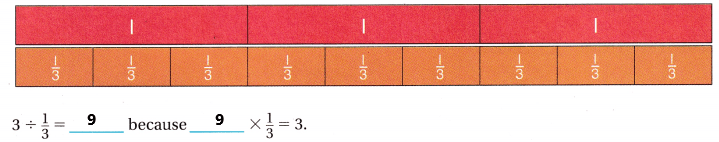Explanation:
In the above image, we can observe 3 units with 3 fraction strips in each unit. Divide the whole number by a fraction. If we divide 3 by 1/3 then the result is 9 because when we multiply 9 with 1/3 the result is 3.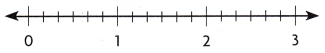Think: What label should I write for each tick mark?
3 ÷ $$\frac{1}{6}$$ = ____________ because ________ × $$\frac{1}{6}$$ = 3.
3 ÷ 1/6 = 18 because 18 × 1/6 = 3.
Explanation:
In the above image, we can observe that the whole number is divided by a fraction. If we divide 3 by 1/6 then the result is 18. Because when we multiply 18 with 1/6 the product is 3. So, the quotient is correct.

Question 3.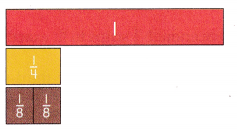$$\frac{1}{4}$$ ÷ 2= ____________ because ________ × 2 = $$\frac{1}{4}$$.
1/4 ÷ 2= 1/8 because 1/8 × 2 =1/4.
Explanation:
In the above image we can observe that 1/4 is divided into 2 equal fraction strips. If we divide 1/4 by 2 then the result is 1/8. Because when we multiply 1/8 with 2 the product is 1/4. So, the quotient is correct.

Problem Solving

H.O.T. Sense or Nonsense?

Question 4.
Emilio and Julia used different ways to find $$\frac{1}{2}$$ ÷ 4. Emilio used a model to find the quotient. Julia used a related multiplication equation to find the quotient. Whose answer makes sense? Whose answer is nonsense? Explain your reasoning.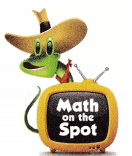Emilio’s Work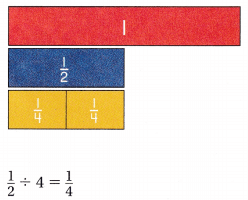Julia’s Work
If $$\frac{1}{2}$$ ÷ 4 = ☐, then ☐ × $$\frac{1}{2}$$
I know that $$\frac{1}{8}$$ × 4 = $$\frac{1}{2}$$
So, $$\frac{1}{2}$$ ÷ 4 = $$\frac{1}{8}$$ because $$\frac{1}{8}$$ × 4 = $$\frac{1}{2}$$
Emilio’s WorkJulia’s Work
If 1/2 ÷ 4 = 1/8, then 1/8 × 4 = 1/2.
I know that 1/8 × 4 = 1/2
So,1/2 ÷ 4 = 1/8 because 1/8 × 4 =1/2.
Explanation:
Emilio and Julia used different ways to find 1/2 ÷ 4. Emilio used a model to find the quotient. Emilio answer is nonsense because when we divide 1/2 by 4 the result is 1/8. But Emilio’s answer is 1/4. Julia used a related multiplication equation to find the quotient. If we divided 1/2 by 4 the result is 1/8 then multiply 1/8 with 4 the result is 1/2. So, Julia’s answer have sense.

For the answer that is nonsense, describe how to find the correct answer.
1/2 ÷ 4 = 1/2 x 1/4 = 1/8
Explanation:
The answer that is nonsense we can find the correct answer by using a related multiplication equation. Divide 1/2 by 4 then the result is 1/8.

Question 6.
Multi-Step If you were going to find $$\frac{1}{2}$$ ÷ 5, explain how you would find the quotient using fraction strips. Write an equation to show the quotient.
Place a strip under a 1/2. We have to find 5 fraction strips, all with the same denominator, that fit exactly under the 1/2 strip.
Each piece is 1/10 of the whole.
Record and check the quotient.
1/2 ÷ 5 = 1/10 because 1/10 × 5 = 1/2.
Explanation:
We can find the quotient using fraction strips. Divide 1/2 by 5 the result is 1/10. The equation is 1/2 ÷ 5 = 1/10.

Question 7.
Ants are lined up along the edges of your sandwich at a picnic. If the sandwich is 9 inches around and each ant is $$\frac{1}{6}$$ of an inch, how many ants are lined up around the sandwich?
(A) 15 ants
(B) 16 ants
(C) 27 ants
(D) 54 ants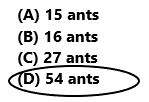9 ÷ 1/6 = 9 x 6/1 = 54
54 ants are lined up around the sandwich.
So, option D is correct.
Explanation:
Ants are lined up along the edges of our sandwich at a picnic. If the sandwich is 9 inches around and each ant is 1/6 of an inch. Divide 9 by 1/6 the result is 54. The ants that are lined up around the sandwich are 54. So, draw a circle to option D.

Question 8.
Use Tools Which is the division problem modeled by the picture?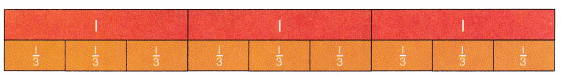(A) 4 ÷ $$\frac{1}{12}$$
(B) 3 ÷ $$\frac{1}{4}$$
(C) 3 ÷ $$\frac{1}{3}$$
(D) 12 ÷ $$\frac{1}{3}$$3 ÷ 1/3 = 9
So, option C is correct.
Explanation:
The division problem that modeled by the above picture is 3 ÷ 1/3. Divide 3 by 1/3 the result is 9. So, draw a circle for option C.

Question 9.
Multi-Step Maddie divided 6 by $$\frac{1}{2}$$, and then divided that answer by $$\frac{1}{3}$$. What was her final answer?
(A) 9
(B) 1
(C) 12
(D) 366 ÷ 1/2 = 12
12 ÷ 1/3 = 36
So, option D is correct.
Explanation:
Maddie divided 6 by 1/2, and then divided that answer by 1/3. First divide 6 by 1/2 the result is 12. Divide 12 by 1/3 the result is 36. The final answer is 36. So, draw a circle for option D.

Texas Test Prep

Reid has 4 bags of soil. He uses $$\frac{1}{3}$$ of a bag to fill each planter. How many planters can Reid fill with the bags of soil?
(A) 1$$\frac{1}{3}$$
(B) 12
(C) 3$$\frac{2}{3}$$
(D) 4$$\frac{1}{3}$$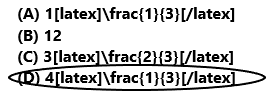Reid can fill 13/3 planters with the bag of soil.
So, option D is correct.
Explanation:
Reid has 4 bags of soil. He uses 1/3 of a bag to fill each planter. 4(1/3) planters can Reid fill with the bag of soil.
So, draw a circle for option D.

### Texas Go Math Grade 5 Lesson 6.4 Homework and Practice Answer Key

Divide and check the quotient.

Question 1.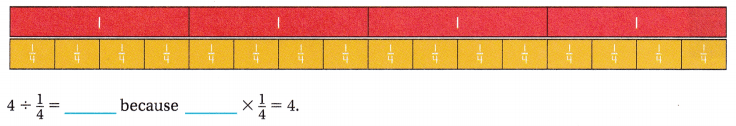Explanation:
In the above image we can observe 4 units with 4 fraction strips in each unit. Divide the whole number by fraction. If we divide 4 by 1/4 then the result is 16 because when we multiply 16 with 1/4 the result is 4.

Question 2.Explanation:
In the above image, we can observe that the whole number is divided by a fraction. If we divide 2 by 1/6 then the result is 12. Because when we multiply 12 with 1/6 the product is 2. So, the quotient is correct.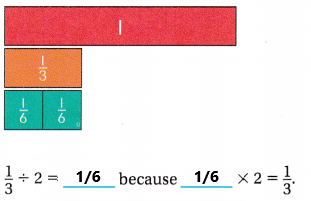Explanation:
In the above image we can observe that 1/3 is divided into 2 equal fraction strips. If we divide 1/3 by 2 then the result is 1/6. Because when we multiply 1/6 with 2 the product is 1/3. So, the quotient is correct.

Question 4.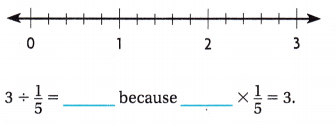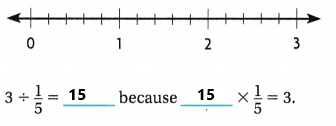Explanation:
In the above image we can observe that whole number is divided by a fraction. If we divide 3 by 1/5 then the result is 15. Because when we multiply 15 with 1/5 the product is 3. So, the quotient is correct.

Question 5.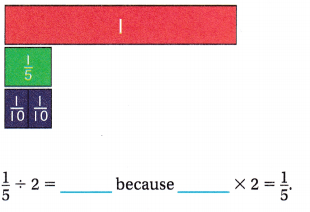Explanation:
In the above image we can observe that 1/5 is divided into 2 equal fraction strips. If we divide 1/5 by 2 then the result is 1/10. Because when we multiply 1/10 with 2 the product is 1/5. So, the quotient is correct.

Problem Solving

Question 6.
Julie buys a board that is 6 feet long. She wants to cut it into pieces that are $$\frac{1}{2}$$ foot each. How many pieces will Julie have after she cuts the board?
6 ÷ 1/2 = 6 x 2/1 = 12
Julie have 12 pieces after she cuts the board.
Explanation:
Julie buys a board that is 6 feet long. She wants to cut it into pieces that are 1/2 foot each. Divide 6 by 1/2 the product is 12. Julie have 12 pieces after she cuts the board.

Mr. Morales makes four batches of cookies for family math night. He divides half of a pound of butter equally into 4 mixing bowls. How much butter is in each bowl?
1/4 x 1/2 = 1/8
In each bowl 1/8 butter.
Explanation:
Mr. Morales makes four batches of cookies for family math night. He divides half of a pound of butter equally into 4 mixing bowls. Divide 1/2 pound of butter into four equal parts. Each part contains 1/4 of butter. Multiply 1/4 with 1/2 the product is 1/8. In each bowl 1/8 butter.

Lesson Check

Question 8.
Which is the division problem modeled by the picture?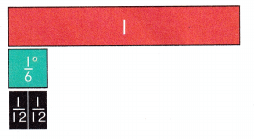(A) $$\frac{1}{2}$$ ÷ 6
(B) $$\frac{1}{6}$$ ÷ 12
(C) $$\frac{1}{6}$$ ÷ 2
(D) $$\frac{1}{12}$$ ÷ 2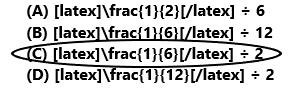Option C is correct.
Explanation:
In the above image we can observe that 1/6 is divided into 2 equal fraction strips. If we divide 1/6 by 2 then the result is 1/12. Because when we multiply 1/12 with 2 the product is 1/6. The division problem modeled by the above picture is 1/6 ÷ 2. So, draw a circle for option C.

Question 9.
Which is the division problem modeled by the picture?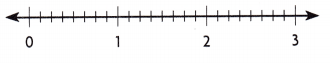(A) 3 ÷ $$\frac{1}{8}$$
(B) 24 ÷ $$\frac{1}{4}$$
(C) 3 ÷ $$\frac{1}{24}$$
(D) 8 ÷ $$\frac{1}{3}$$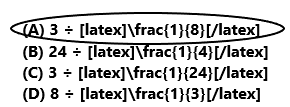Option A is correct.
Explanation:
In the above image we get the information by observing the number line. The whole number is divided by a fraction. If we divide 3 by 1/8 then the result is 24. Because when we multiply 24 with 1/8 the product is 3. The division problem modeled by the above picture is 3 ÷ 1/8. So, draw a circle for option A.

Evan brings 8 liters of juice to be served at family math night. He pours 1/5 liter into each glass. How many glasses of juice can be served?
(A) 8
(B) 40
(C) 13
(D) 10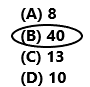8 ÷ 1/5 = 8 x 5/1 = 40
40 glasses of juice can be served at family math night.
So, option B is correct.
Explanation:
Evan brings 8 liters of juice to be served at family math night. He pours 1/5 liter into each glass. Divide 8 by 1/5 the result is 40. At family math night 40 glasses of juice can be served. So, draw a circle for option B.

Question 11.
Marissa is painting one of her bedroom walls. She marks off $$\frac{1}{4}$$ of the wall. Then she divides the marked section into 3 equal parts and paints one part blue. What fraction of the whole wall is blue?
(A) $$\frac{1}{3}$$
(B) $$\frac{1}{4}$$
(C) $$\frac{1}{7}$$
(D) $$\frac{1}{12}$$1/4 ÷ 3 = 1/4 x 1/3 = 1/12
1/12 fraction of the whole wall is blue.
So, option D is correct.
Explanation:
Marissa is painting one of her bedroom walls. She marks off 1/4 of the wall. Then she divides the marked section into 3 equal parts and paints one part blue. Divide 1/4 by 3 the result is 1/12. 1/12 fraction of the whole wall is blue. So, option D is correct.

Question 12.
Multi-Step Rex combines $$\frac{1}{4}$$ cup sugar and 1/4 cup flour Into a small bowl. Then he divides the amount in the small bowl into 4 empty mixing bowls. What amount of dry Ingredients is in each mixing bowl?
(A) $$\frac{1}{16}$$ cup
(B) $$\frac{1}{2}$$ cup
(C) $$\frac{1}{8}$$ cup
(D) 2 cups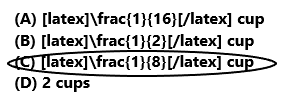1/4 + 1/4 = 1/2
1/2 ÷ 4 = 1/8
In each mixing bowl 1/8 amount of dry ingredients.
So, option C is correct.
Explanation:
Rex combines 1/4 cup sugar and 1/4 cup flour Into a small bowl. Add 1/4 with 1/4 the sum is 1/2. Then he divides the amount in the small bowl into 4 empty mixing bowls. Divide 1/2 by 4 the result is 1/8. In each mixing bowl 1/8 amount of dry ingredients. So, draw a circle for option C.

Multi-Step Jamie divided 8 by $$\frac{1}{2}$$, and then multiplied that answer by $$\frac{1}{4}$$. What was her final answer?
(A) 4
(B) 1
(C) 64
(D) 32